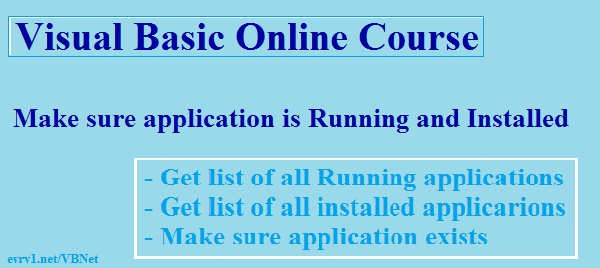## Search VB 2010

Showing posts with label Visual Basic .net. Show all posts
Showing posts with label Visual Basic .net. Show all posts

##Visual Basic Online Course - Tips

When you create a Visual Basic 2010 or generally a VB.Net application that relies on another VB.Net application for example an Updater.exe application, you will have to code both sides in order no to enable the user to run Updater.exe without the existence of the Main application, and in order to do so, you will have to make sure that :
1) Your Main application is Running.
2) Your Main application is Installed.
3) Your Main application does exist in the installation folder.
After making sure of all the above points, then you can compile your updater.exe file with your Main Application knowing that updater.exe won't run alone, especially if you're sending updates to the Main application for the user to receive using Updater.exe.

Here are the code to do so :
1) VB 2010 Get list of all installed applications

2) VB 2010 Get list of all running applications

3) VB 2010 Make sure application exists

## Microsoft Visual Basic 2010

Math Functions
Math and String Functions
In addition to performing simple arithmetic and string operations with the arithmetic and string operators, Visual Basic programs can take advantage of several built-in mathematical functions and string functions to perform useful processing that, otherwise, could require highly complex original code.
Mathematical Functions
Popular mathematical functions are summarized in the following table. Note that certain functions do not require the Math. prefix.
FunctionUse
Math.Abs()Returns the absolute value.
Math.Abs(-10) returns 10.
Math.Ceiling()Returns an integer that is greater than or equal to a number.
Math.Ceiling(5.333) returns 6.
Fix()Returns the integer portion of a number.
Fix(5.3333) returns 5.
Math.Floor()Returns an integer that is less than or equal to a number.
Fix(5.3333) returns 5.
Int()Returns the integer portion of a number.
Int(5.3333) returns 5.
Math.Max()Returns the larger of two numbers.
Math.Max(5,7) returns 7.
Math.Min()Returns the smaller of two numbers.
Math.Min(5,7) returns 5.
Math.Pow()Returns a number raised to a power.
Math.Pow(12,2) returns 144.
Rnd()Returns a random number between 0 and 1. Used in conjunction with Randomizestatement to initialize the random number generator.
Math.Round()Rounds a number to a specified number of decimal places. Rounds up on .5.
Math.Round(1.1234567,5) returns 1.12346.
Math.Sign()Returns the sign of a number. Returns -1 if negative and 1 if positive.
Math.Sign(-5) returns -1.
Math.Sqrt()Returns the square root of a positive number.
Math.Sqrt(144) returns 12.
Random Numbers
The Rnd() function returns a random number between 0 and 1. More likely, the need is to generate a number within a particular range, between a given low and high number. This is accomplished with the following formula.
 Math.floor((high - low + 1) * Rnd() + low)
For instance, to generate a random number between 0 and 10 the formula becomes
`Math.floor((10 - 0 + 1) * Rnd() + 0)`
String Functions
Several built-in string functions perform string manipulations to augment simple concatenation with the "&" operator. These functions are summarized in the following table.
FunctionUse
Asc()Returns the character code of the first character of a string.
Asc("A") returns 65.
Chr()Returns the display character of a character code.
Chr(65) returns "A".
GetChar()Returns the character at a specified position in a string, counting from 1.
GetChar("This is a string", 7) returns "s".
InStr()Returns the starting position in a string of a substring, counting from 1.
InStr("This is a string", "string") returns 11.
InStrRev()Returns the starting position in a string of a substring, searching from the end of the string.
InStr("This is a string", "string") returns 11.
LCase()Returns the lower-case conversion of a string.
LCase("THIS IS A STRING") returns "this is a string".
Left()Returns the left-most specified number of characters of a string.
Left("This is a string", 4) returns "This".
Len()Returns the length of a string.
Len("This is a string") returns 16.
LTrim()Removes any leading spaces from a string.
LTrim("   This is a string") returns "This is a string".
Mid()Returns a substring from a string, specified as the starting position (counting from 1) and the number of characters.
Mid("This is a string", 6, 4) returns "is a".
Replace()Replaces all occurrences of a sub-string in a string.
Replace("This is a string", " s", " longer s") returns "This are a longer string" (replaces an "s" preceded by a blank space).
Right()Returns the right-most specified number of characters of a string.
Right("This is a string", 6) returns "string".
RTrim()Removes any trailing spaces from a string.
RTrim("This is a string   ") returns "This is a string".
Str()Returns the string equivalent of a number.
Str(100) returns "100".
Space()Fills a string with a given number of spaces.
"This" & Space(5) & "string" returns "This     string".
StrComp()Compares two strings. Return values are 0 (strings are equal), 1 (first string has the greater value), or -1 (second string has the greater value) based on sorting sequence.
StrComp("This is a string", "This string") returns -1.
StrReverse()Reverses the characters in a string.
StrReverse("This is a string") returns "gnirts a si sihT".
Trim()Removes any leading and trailing spaces from a string.
Trim("   This is a string   ") returns "This is a string".
UCase()Returns the upper-case conversion of a string.
UCase("This is a string") returns "THIS IS A STRING".
Val()Converts a numeric expression to a number.
Val( (1 + 2 + 3)^2 ) returns 36.
The above summaries give you a basic idea of the uses of these arithmetic and string functions. There are occasions throughout these tutorials to see them in action and in combination as they are applied to various processing needs.